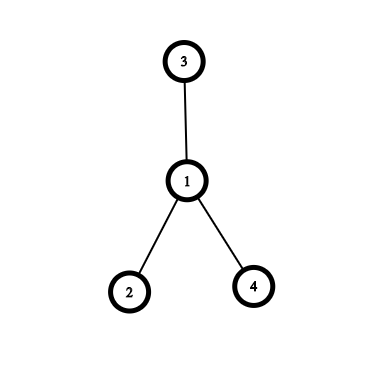# Lost in Binary TreerebornIntra UAP Programming Con...
Limits 1s, 128 MB ·

You have a rooted unweighted binary tree. But somehow you forgot which vertex was the root. Well, you can ask me. I remember. But let's play a game. In each move, you can pick a vertex and ask me the distance of that vertex from that root. And I'll tell you so. However, I'll answer no more than $20$ of your queries.

## Input

The first line of input will contain an integer $N$ denoting the number of vertices. Following $N - 1$ lines will describe the tree. Each line will have two space-separated integers $u, ~v$ representing an edge between $u$ and $v$.

• $1 \le N \le 10^5$.
• Vertices are numbered from $1$ to $N$.
• You can be assured that the input will describe a valid tree.

### Interaction

To ask a question, that is to ask how far a vertex $v$ is from root, print "? $v$" without the quotes in a single line and flush the output. The judge will reply with an integer $d$ as the answer to your query. After you have figured out the root vertex $r$, you can submit your answer via printing "! $r$" without quotes in a single line.

After submitting the root properly, your program must quit immediately since the interactor will stop interaction and depending upon the submission you'll receive "Accepted" or "Wrong answer". If you have asked more than $20$ questions or any of your output format is wrong, the interactor will output an integer value of $-1$ and will stop interaction immediately. At any point after receiving $-1$, Your program must quit immediately and will receive "Wrong answer".

Note that submitting the root $r$ is not counted as one of the $20$ queries.

### Sample I/O

> 4
> 1 2
> 1 3
> 1 4
< ? 1
> 1
< ? 2
> 2
< ! 3


> indicates what your program reads and < indicates what your program writes. These symbols are here to make it easy to understand. You must not print such symbols from your program.The above figure describes the tree from input in line 1 to 4 in Sample I/O.

• Upon asking the distance from 1 in line 5, the interactor replies with 1 in line 6.
• Upon asking the distance from 2 in line 7, the interactor replies with 2 in line 8.
• Upon submitting the root as 3 in line 9, the interactor quits immediately.

### Notes

Since this is an interactive problem, don’t forget to flush your output after printing each line. You may use fflush(stdout) for printf/cout in C/C++, import sys and sys.stdout.flush() in Python to flush the output. If you use some other programming language, consult it's documentation.Interactive

### Submit